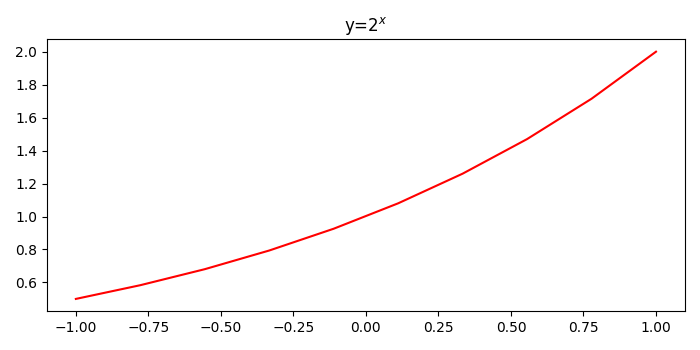# How to add a variable to Python plt.title?

To add a varaible to Python plt.title(), we can take the following steps −

• Create data points for x and y using numpy and num (is a variable) to calculate y and set this in title.

• Plot x and y data points using plot() method with red color.

• Set the title of the curve with variable num.

• To display the figure, use show() method.

## Example

import numpy as np
from matplotlib import pyplot as plt
plt.rcParams["figure.figsize"] = [7.00, 3.50]
plt.rcParams["figure.autolayout"] = True
x = np.linspace(-1, 1, 10)
num = 2
y = num ** x
plt.plot(x, y, c='red')
plt.title(f"y=%d$^x$" % num)
plt.show()

## Output